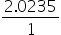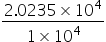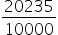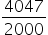Maths-
General
Easy

Question

# Convert the decimal into a fraction: 2.0325Hint:

## The correct answer is: 4047/2000

### a)Step 1 of 3:Write down 2.0235 divided by 1:=Step 2 of 2:Multiplying numerator and denominator by 104(because there are 4 digits after the decimal point)==Step 2 of 3:Simplifying the fraction=Final Answer: Hence, the fraction of 2.0235 is.#### With Turito Foundation.#### Get an Expert Advice From Turito.